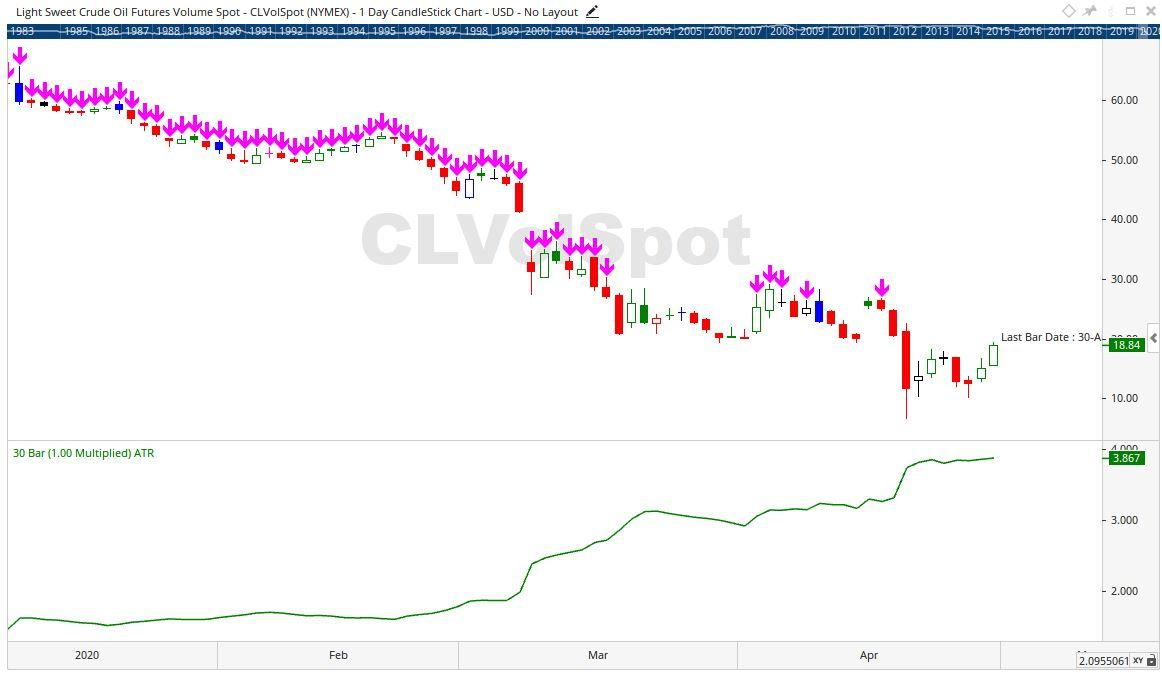Select Page

# Reply To: ATR% of Close Price

Optuma Forums Optuma Scripting ATR% of Close Price Reply To: ATR% of Close Price

#57903

I suspect your premise will nearly always be achieved.

You are calculating the Average True Range of the movement of the Close price of a group of bars, which is always significantly smaller than the Close prices, and looking for that value to be less than 15% of a Close price.

As a couple of  examples:

• Sugar (SBVolSpot) at the present ATR(30) = 0.393, while the Close price in 10.37, 15% of which is 1.55.
• SPI 200 Index (APCVolSpot) at present ATR(30) = 224.186, while the Close price in 5,539.00, 15% of which is 830.85.

The only exception I could find was the recent extreme movement in Crude Oil which came up with this result on 30 Apr 2020: ATR(30) = 3.867, while the Close price in 18.84, 15% of which is 2.826.I’d also recommend bracketing your formula to ensure the correct comparison sequence of calculation:

(ATR(BARS=10)/CLOSE()) < (15/100)

I’d also simplify your formula to save an unnecessary calculation::

(ATR(BARS=10)/CLOSE()) < 0.15

Cheers

Trevor